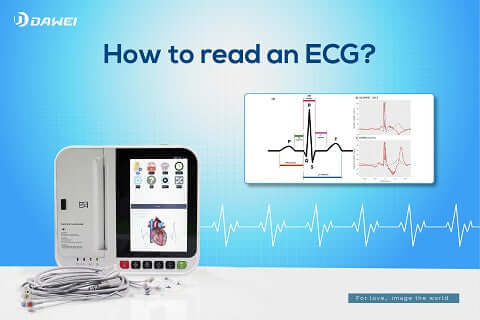Casa / Blog / How to read an ECG? EKG/Electrocardiogram# How to read an ECG? EKG/Electrocardiogram

ECG physical examination is of great value in routine clinical examination, so it is extremely necessary for clinicians to master the interpretation of ECG.
How to read an ECG? Let's start by understanding the meaning of the ECG waveform and the associated measurement information.
One of the most important steps in reading an ECG is to measure the individual ECG waveforms to see if they are normal.
The complete beating cycle of an ECG can be fully demonstrated by six waveforms, P-Q-R-S-T-U, with two dimensions of time and gain.
The main measurements of ECG include two major components: time and voltage amplitude.
(1) Transverse spacing represents time, 0.04 s per small frame and 0.20 s per large frame.
(2) Longitudinal is the voltage amplitude, standard voltage 1mv=10 mm, longitudinal spacing is equal to 0.1mV per small frame, 0.5mV per large frame
1. P wave
P wave represents the sinus node giving pacing point command, sinus P wave; PP interval is one complete heartbeat cycle, usually one electrical command can appear one P wave and one QRS wave, for the convenience of measurement, RR interval is usually used instead of PP interval; it is used to assess the autoregulation of sinus node; i.e. the number of heartbeats per minute.
Shape:obtuse round
Direction:I, II, aVF upward, aVR downward
Amplitude: limb conduction <0.25 mv="" chest="" conduction="" 0="" 20="" div="" data-mce-fragment="1">
Time limit:≤0.12s
1. PR interval
Represents the conduction process from the sinoatrial node to the AV node.
Time: normal range 120-200ms, >200ms means decreased AV node conduction function
1. QRS wave interval
The depolarization wave of the right and left ventricles, representing the bundle branch conduction function.
Time:0.06~0.10s
Amplitude:RV1 ≤ 1.0 mv, in complete right bundle branch block RV1 ≤ 1.5 mv; RaVR < 0.5 mv; RV5, RV6 ≤ 2.5 mv; RI < 1.5 mv; RaVL < 1.2mv; RaVF < 2.0mv
Abnormal Q wave: the amplitude of Q wave is greater than 1/4 of the amplitude of the R wave in the same lead, the time limit ≥ 0.04s
1. ST segment
Ventricular slow repolarization, used to judge the function of myocardial reset, measured by the zero line (i.e. PR line), generally -0.05 -0.1mv.
Upward shift: <0.1 mv="" v4="" v6="" leads="" 0="" 5="" v3="" 3="" v1="" v2="" div="" data-mce-fragment="1">
Time limit:0.05~0.15s
1. T wave
Ventricular rapid repolarization.
Direction: The direction of the T wave is mostly the same as the direction of the main QRS wave, the T wave is up in leads I, II, V4~V6, down in aVR, up or down in leads V1~V3, but if it is upright in lead V1, it should not be inverted in leads V2 and V3.
Amplitude: not less than 1/10 of the R wave in the same lead
Time limit:<0.25 s="" div="" data-mce-fragment="1">
1. QT interval
It is the total time of cardiac depolarization and repolarization process. A long QT interval may induce malignant arrhythmia.
Time:0.32~0.44 s, female <0.46 s="" male="" 0="" 45="" ventricular="" rate:="" 60="" 100="" bpm="" qtc="" 44s="" corrected="" qt="" interval="" div="" data-mce-fragment="1">
1. U wave
The effect of the ventricular relay potential, a wavelet generated after the T wave.
Direction: direction is generally consistent with T wave, V3 is obvious; amplitude does not exceed 1/2 of the T wave in the same lead
Increased: hypokalemia, hypercalcemia, drug effects, acute cerebrovascular accident, etc.
Inversion: suggests anterior descending branch lesion, hypertension, right ventricular hypertrophy or overload.